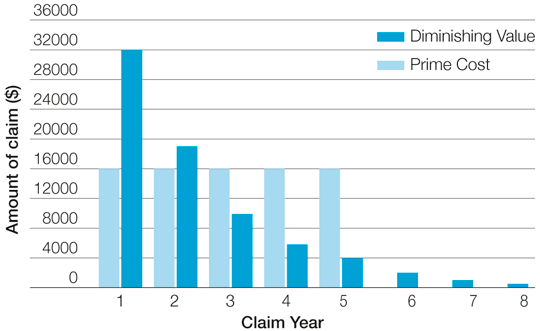• # Prime cost (straight line) and diminishing value methods

In most cases, you can choose to use either of two alternative methods for calculating depreciation:

• The prime cost method assumes that the value of a depreciating asset decreases uniformly over its effective life.
• The diminishing value method assumes that the value of a depreciating asset decreases more in the early years of its effective life.

To calculate depreciation for most assets for a particular income year, you can use the Depreciation and capital allowances tool, which compares results of the two methods and also provides disposal outcomes.

This graph compares the amount you would claim under each method for the depreciation of an asset that is used only for business. The asset in this example cost \$80,000, was acquired on the first day of the income year and has an effective life of five years.## Prime cost (straight line) method

Under the prime cost method (also known as the straight-line method), you claim a fixed amount each year based on the following formula:

Asset’s cost × (days held ÷ 365) × (100% ÷ asset’s effective life)

Note: ‘Days held’ is the number of days you held the asset in the income year in which you used it or had it installed ready for use for any purpose. Days held can be 366 for a leap year.

Example 1: Prime cost method

If the asset costs \$80,000 (after excluding GST if entitled to claim it) and has an effective life of five years, you can claim 20% of its cost, or \$16,000, in each of the five years.

The cost includes the amount you paid for the asset as well as any additional amounts paid for transport, installation or making it ready to use.

The calculation is:

\$80,000 × (365 ÷ 365) × 20% = \$16,000

End of example

Note that if you acquired the above asset part way through the year, the final calculation using the prime cost method should occur in the sixth year for the remaining portion that was not claimed in the first year.

## Diminishing value method

The following formula is used for the diminishing value method:

Base value × (days held ÷ 365) × (200% ÷ asset’s effective life)

Days held can be 366 for a leap year. (see Note)

Example 2: Diminishing value method

If the asset cost \$80,000 and has an effective life of five years, the claim for the first year will be:

\$80,000 × (365 ÷ 365) × (200% ÷ 5) = \$80,000 × 40% = \$32,000

The cost includes the amount you paid for the asset (excluding GST if entitled to claim it) as well as any additional amounts paid for transport, installation or making it ready to use.

The base value reduces each year by the decline in the value of the asset. This means the base value for the second year will be \$48,000; that is, \$80,000 minus the \$32,000 decline in value in the first year.

The claim for the second year will be:

\$48,000 × (365 ÷ 365) × (200% ÷ 5) = \$48,000 × 40% = \$19,200

In the third year, the base value will be \$28,800 and the claim will be \$11,520.

In the fourth year, the base value will be \$17,280 and the claim will be \$6,912.

This will continue until the value reaches zero.

End of example

If you started to hold the asset before 10 May 2006, the formula for the diminishing value method is:

Base value × (days held ÷ 365) × (150% ÷ asset’s effective life)

## Reduction for non-taxable use

Irrespective of the method used, a deduction for the decline in value of a depreciating asset is reduced by the extent to which it's used for a non-taxable purpose. For example, if an asset is used 40% of the time for a private purpose, the deduction for its decline in value is reduced by 40%. Hence all figures above would be multiplied by a factor of 0.6.

## Transfer to low-value pool

Once the value of the asset falls below \$1,000, you can choose to transfer its remaining value to a low-value pool. By doing so, you can claim depreciation for the asset together with any other low-value assets, rather than making separate calculations for each.

## Backing business investment – accelerated depreciation

For assets first held on or after 12 March 2020 and first used or installed ready for use until 30 June 2021 the Backing business investment measure provides a time-limited investment incentive to support business investment and economic growth by accelerating depreciation deductions. The key features of the incentive are as follows:

• The benefits are either
• Deduction of 50% of the cost or opening adjustable value of an eligible asset on installation. Existing depreciation rules apply to the balance of the asset’s cost.
• If you are using the simplified depreciation rules for small business you can claim 57.5% of the cost of the asset in the first year you add the asset to the small business pool.